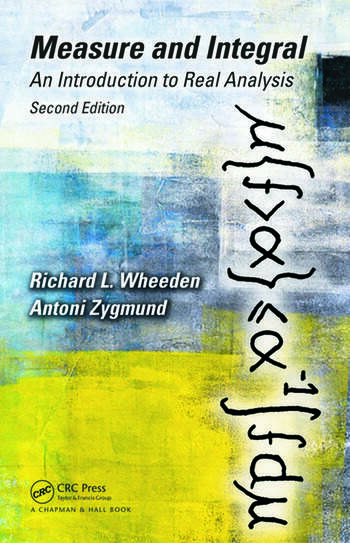# Measure and Integral: An Introduction to Real Analysis, Second Edition

## 2nd Edition

Richard L. Wheeden

Chapman and Hall/CRC
Published April 24, 2015
Textbook - 532 Pages - 8 B/W Illustrations
ISBN 9781498702898 - CAT# K24634
Series: Chapman & Hall/CRC Pure and Applied Mathematics

For Instructors Request Inspection Copy

was \$120.00

USD\$96.00

SAVE ~\$24.00

FREE Standard Shipping!

## Preview

### Summary

Now considered a classic text on the topic, Measure and Integral: An Introduction to Real Analysis provides an introduction to real analysis by first developing the theory of measure and integration in the simple setting of Euclidean space, and then presenting a more general treatment based on abstract notions characterized by axioms and with less geometric content.

Published nearly forty years after the first edition, this long-awaited Second Edition also:

• Studies the Fourier transform of functions in the spaces L1, L2, and Lp, 1 < p < 2
• Shows the Hilbert transform to be a bounded operator on L2, as an application of the L2 theory of the Fourier transform in the one-dimensional case
• Covers fractional integration and some topics related to mean oscillation properties of functions, such as the classes of Hölder continuous functions and the space of functions of bounded mean oscillation
• Derives a subrepresentation formula, which in higher dimensions plays a role roughly similar to the one played by the fundamental theorem of calculus in one dimension
• Extends the subrepresentation formula derived for smooth functions to functions with a weak gradient
• Applies the norm estimates derived for fractional integral operators to obtain local and global first-order Poincaré–Sobolev inequalities, including endpoint cases
• Proves the existence of a tangent plane to the graph of a Lipschitz function of several variables
• Includes many new exercises not present in the first edition

This widely used and highly respected text for upper-division undergraduate and first-year graduate students of mathematics, statistics, probability, or engineering is revised for a new generation of students and instructors. The book also serves as a handy reference for professional mathematicians.

#### Instructors

We provide complimentary e-inspection copies of primary textbooks to instructors considering our books for course adoption.